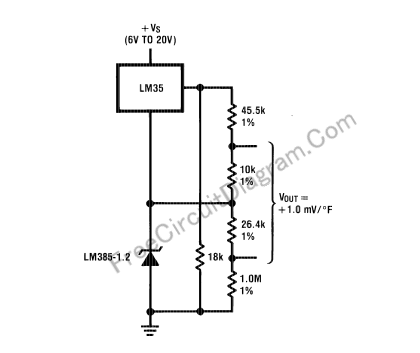# Fahrenheit Thermometer Using LM35 Temperature Sensor ICIf you have a digital voltmeter, or any voltmeter with millivolt resolution and high input impedance, then you can use this temperature-to-voltage adapter circuit to measure room temperature. Here is the circuit’s schematic diagram:Note that the voltage output of this circuit is floating, not referenced to ground. You have to use separate supply if your voltmeter has single ended (referenced to ground) input. Differential input adapter described here is suitable to give a differential input for your single ended meter, remember that the input impedance must be high. You can set your voltmeter to 200 mV range to give temperature reading directly in Fahrenheit degree. [Circuit’s schematic source: National Semiconductor Application Notes]